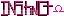-=+=- -=+=- -=+=- -=+=- -=+=- -=+=- -=+=- -=+=- -=+=- -=+=- -=+=- -=+=- -=+=- -=+=- -=+=- -=+=- -=+=- -=+=- -=+=- -=+=- -=+=- -=+=- -=+=- -=+=- -=+=- -=+=- -=+=- -=+=- -=+=- -=+=- (c) WidthPadding Industries 1987 0|533|0 -=+=- -=+=- -=+=- -=+=- -=+=- -=+=- -=+=- -=+=- -=+=- -=+=- -=+=- -=+=- -=+=- -=+=- -=+=- -=+=- -=+=- -=+=- -=+=- -=+=- -=+=- -=+=- -=+=- -=+=- -=+=- -=+=- -=+=- -=+=- -=+=- -=+=-
SoCoder -> Article Home -> Coding Basics

SchererererCreated : 15 September 2006
Edited : 15 September 2006

### Hello, World in .Net

Hello world in VB or C#.Net

.NET is great! If you have no idea what it is, check this out, and this aswell. I'm going to give you a quick tutorial on how to make a hello world console program in C# and VB. Both languages use the .Net framework so its easy to put them both in one tutorial. I'll be using MS Visual Studio 2005 .Net.

You can get a free version of MS Visual Studio 2005 Express Edition from here. J#.Net is also available, but next to no one uses it. If you're already comfortable with java you might want to use it, but C# is just like it except some keywords are changed and also its got a lot more goodies such as operator overloading and better support for generics, but we're not getting into that in this tutorial, just hello world.

Ok, so first things first, download the .Net IDE of your choice (VB or C#). Open it up; it'll want to do some registration, so click through it real quick and play nice. Now you are going to want to create a new solution. A solution is basically a project file, Microsoft just thought they were being clever when they called it a solution. Select "Console Application" and it will create a file called Program.cs in C# and Module1.vb in Visual Basic. This is what you should get in C#:

... and in VB

ok, ok, ok, don't get scareed yet. In the C# one it talks about these namespaces, don't worry, its just a method of grouping a lot of your .cs files. Also in the C# it defines a class called Program. This is where all the magic happens, you can put fields and define methods in this area. A method that was created automatically was the Main() method. its defined as static because it stays the same all the time, and void because we don't need to return anything. If you don't know what i mean, then don't worry, you're still ok. Inside the parenthasis it has string[] args. this gets any command-line arguments that you might want to include. You don't need this though for what we're doing.

Now we'll look at the VB code. It defines a Module, which is kinda like the class we defined in the C# code. And then it makes a Sub called Main(), which does the same thing as the C# Main() function.

Let's add some stuff to Main(). Main is what's executed when the program runs. If you hit the little play button at the top you'd notice that a console window comes up and flashes... that's because there's nothing in there. I'll give you the code to look at and then we'll go over what i did.

Woah, looks like i wrote the exact same code in both places, except the C# one has semi colons. These are necessary to end every line of C#; i'll describe its advantages in another article. let's go over what each one is doing, although it should be fairly self-explanitory.

Console.WriteLine("Hello, World!")

This line basically takes the string "Hello, World!" and prints it to the console window. The quotes are necessary because they group the letters together.

Console.Read();

Technically, what this is doing is reading a character that you input. However, we're using it to pause the program so that you can see the text. If we took it out the console window would appear with the text so fast you wouldn't be able to read it.

looks like we're done here! I plan on writing more articles outlining the finer points of .Net and OOP, and also comparing Java and C# where they both rise and fall.

### Comments

Friday, 15 September 2006, 21:00
magicmanand in java....

somthing like that at least. might need to capitalize a few diffent things.Saturday, 16 September 2006, 11:17
SchererererCorrect, here's a more complete list of ALL hello, world programs: en.wikipedia.org/wiki/List_of_hello_world_programs